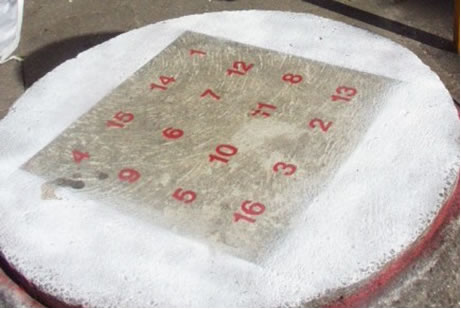#### You may also like### Prompt Cards

These two group activities use mathematical reasoning - one is numerical, one geometric.### Consecutive Numbers

An investigation involving adding and subtracting sets of consecutive numbers. Lots to find out, lots to explore.### Exploring Wild & Wonderful Number Patterns

EWWNP means Exploring Wild and Wonderful Number Patterns Created by Yourself! Investigate what happens if we create number patterns using some simple rules.

# All Seated

##### Age 7 to 11Challenge Level

Found in the playground of a Danish school - something to sit on!Look carefully at the numbers.

What do you notice?

Can you make another square using the numbers $1$ to $16$, that displays the same properties?

Find out what Albrecht DÃ¼rer has to do with this arrangement of numbers.

There are twelve different types of $4$ by $4$ magic square that use the numbers $1$ to $16$. See if you can find information about these twelve types and show examples of each one.

Look carefully in each case at the patterns produced when a total of $17$ is searched for.

Can you finish off the $5$ by $5$ magic square below?

 $23$ $6$ $19$ $2$ $15$ $10$ $1$ $5$ $9$ $4$ $12$ $8$ $11$ $7$ $3$